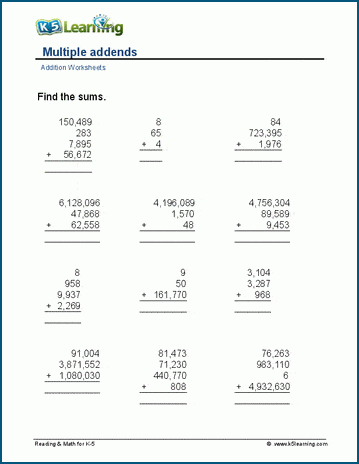# Multi-digit Addition Math Drill Worksheets

In this section of multi-digit addition worksheets, the emphasis is on building skills for the addition of large numbers in columns, with and without regrouping.

Let’s take you through the sub-sections.

There are three sets of worksheets in this area: addition tables 11-20, 11-50 and 11-100.

This is one of the 11-50 addition tables worksheets: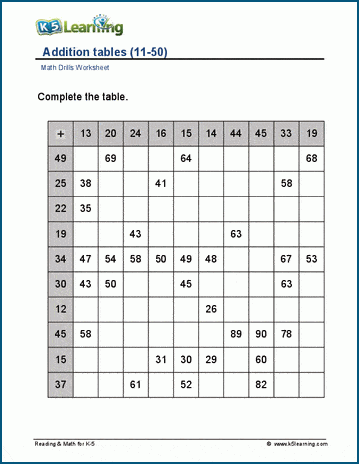## Addition in columns with no regrouping worksheets

Students who want to practice adding 2- and 3-digit numbers with no regrouping will want to use the worksheets in this sub-section. Each page contains worksheets with 25 and 42 questions, depending on how many you want to drill.

This worksheet covers 3-digit plus 2-digit addition with no regrouping.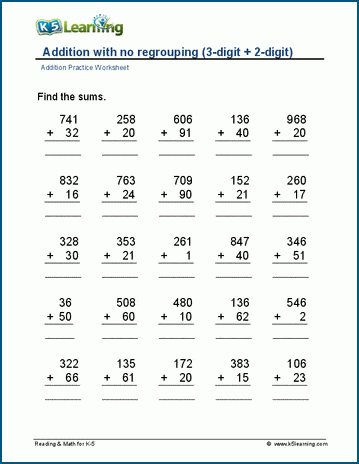## Addition in columns with regrouping

This next section is larger covering multiple regrouping multi-digit addition worksheets, including: addition with sums up to 50, addition with sums up under 100, and 2-digit up to 7-digit addition. As with the above section, each page contains worksheets of 25 and 42 questions.

Here’s what the 4-digit plus 3-digit worksheets looks like: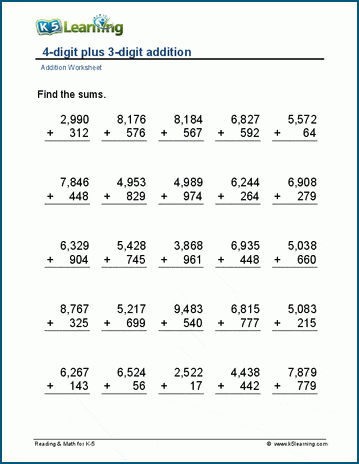## Horizontal addition of 2- and 3-digit numbers

Adding multi-digits horizontally presents a new way for students to work on the answers, often getting students to calculate large numbers mentally.

This worksheet has students work on sums under 100.

https://www.k5learning.com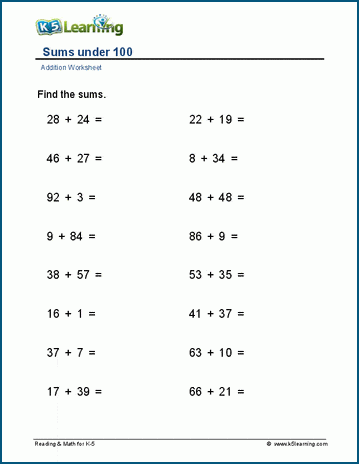## Adding whole tens, hundreds and thousands

These worksheets cover adding whole tens, hundreds and thousands, as well as worksheets where the numbers added make 100 or make 1,000. It’s another larger sub-section with many different worksheets.

In this worksheet students add up to 4 addends of tens, hundreds and thousands.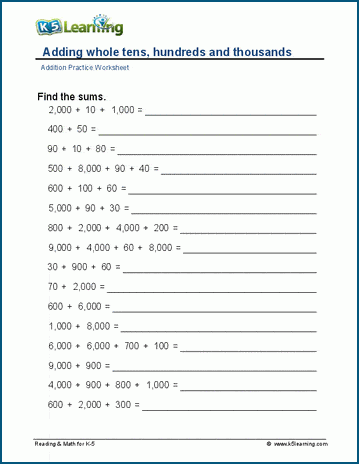This sub-section covers worksheets where there’s a missing addend in 2- and 3-digit addition.

In this worksheet, students are working on missing addends in 2-digit addition: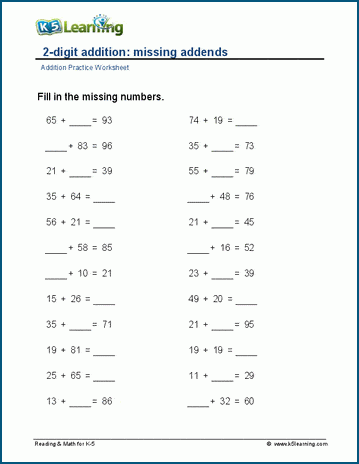## Practice 3 or more addends

The final sub-section covers worksheets on three to four addends in various 2-, 3-, 4-, 5-, and 6-digit worksheets.

At the end, we have two sets of mixed practice worksheets. This is what one of the mixed practice worksheets looks like: